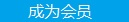您可以捐助，支持我们的公益事业。 1元 10元 50元 认证码：必填求知 文章 文库 Lib 视频 Code iProcess 课程 角色 咨询 工具 火云堂 讲座吧 成长之路要资料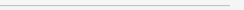订阅捐助
C++11之美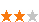1500 次浏览     评价： 好 中 差

C++11/14相比以往的C++98/03在很多方面做了简化和增强，尤其是在泛型编程方面，让C++的泛型编程的威力变得更加强大，本文将用三个案例来介绍C++11相比C++98/03如何以一种更灵活、更简洁和更泛化的方式去解决问题。

 `template void foo(const T& t) { if (t has foo) t.foo(); else default(); } `

 `template struct has_member_foo { template struct SFINAE {}; template static char check(SFINAE*); template static int check(...); static const bool value = sizeof(check(0)) == sizeof(char); }; `

 `struct MyStruct { void foo() { } }; static_assert(has_member_foo::value, "true"); //断言通过 static_assert(has_member_foo::value, "false"); //断言错误 `

C++11的实现方式是这样的。

 `template struct has_member_foo11 { private: template static auto check(int) -> decltype(std::declval().foo(), std::true_type()); template static std::false_type check(...); public: enum { value = std::is_same(0)), std::true_type>::value }; }; `

C++11的实现不需要辅助结构体，用到了几个C++11的新特性：auot、decltype、返回类型后置、declval和trype_traits。具体的实现原理是这样的：通过返回类型后置来推断表达式的类型，推断的过程中利用declval，它可以获取类型的右值引用，以便来调用成员函数，这个过程是在编译期完成的。如果通过declval调用成员函数成功了，则会继续推断逗号表达式的类型，最终推断的函数返回类型为std::true_type；如果通过declval调用成员函数失败了则推断失败，编译器会选择优先级最低的check(...)函数，它的返回类型为std::false_type。我们最后根据返回类型既可知道类型是否存在foo函数。

 `struct MyStruct { int foo() { } }; static_assert(has_member_foo::value, "false"); //断言错误 static_assert(has_member_foo11::value, "true"); `

 `template struct has_member_foo11 { private: template static auto check(int) -> decltype(std::declval().foo(std::declval()...), std::true_type()); template static std::false_type check(...); public: enum { value = std::is_same(0)), std::true_type>::value }; }; `

 `struct MyStruct { string foo() { return ""; } int foo(int i) { return i; } }; static_assert(has_member_foo::value, "true"); static_assert(has_member_foo::value, "true");`

 `#define HAS_MEMBER(member)\ templatestruct has_member_##member\ {\ private:\ template static auto Check(int) -> decltype(std::declval().member(std::declval()...), std::true_type());\ template static std::false_type Check(...);\ public:\ enum{value = std::is_same(0)), std::true_type>::value};\ }; `

 `HAS_MEMBER(foo) HAS_MEMBER(func)`

 `struct MyStruct { string foo() { return ""; } int func(int i) { return i; } }; static_assert(has_member_foo::value, "true"); static_assert(has_member_func::value, "true"); `

 `template typename std::enable_if::value>::type testfun(T t) { cout << "has foo" << endl; } template typename std::enable_if::value>::type testfun(T t) { cout << "has no foo" << endl; } `

 `size_t fibonacci(size_t n) { return (n < 2) ? n : fibonacci(n - 1) + fibonacci(n - 2); } void test() { Timer t; size_t n = fibonacci(45); cout << n << " " << t.elapsed() << endl; } `

 `size_t fibonacci(size_t n) { return (n < 2) ? n : sugar(fibonacci2)(n - 1) + sugar(fibonacci2)(n - 2); } `

 `template std::function sugar(R(*func)(Args...), bool needClear = false) { using function_type = std::function; static std::unordered_map functor_map; if (needClear) return functor_map[func] = cache(func); if (functor_map.find(func) == functor_map.end()) functor_map[func] = cache(func); return functor_map[func]; } `

sugar函数内部有一个map（注意，这里用的静态局部变量，C++11中的静态局部变量是线程安全的），用来保存被缓存函数的函数指针和一个std::function，std::function又是从cache函数返回出来的，接下来看看cache的实现。

 `template std::function cache(R(*func) (Args...)) { auto result_map = std::make_shared, R>>(); return ([=](Args... args){ std::tuple t(args...); if (result_map->find(t) == result_map->end()) (*result_map)[t] = func(args...); return (*result_map)[t]; }); } `

cache内部有一个map，它保存了被缓存函数调用时的入参和函数的执行结果。cache函数还定义了一个lambda表达式，用于延迟执行，在外面调用function时才执行。Lambda内部的逻辑很简单就是保存调用时的参数和执行结果，当下次调用时会先检查调用的参数是否已经存在，如果已经存在则从缓存map中返回，如果不存在才调用被缓存的函数，同时保存新的调用参数和调用结果。图1是fibonacci函数缓存的过程。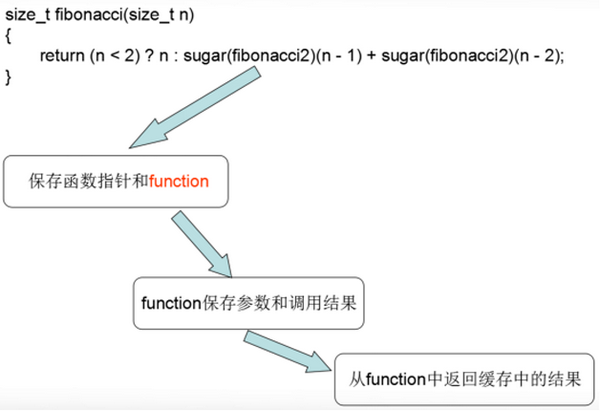C++11实现的这个函数缓存相比传统的缓存方法来说有下面几个优点：

1．相比传统的缓存实现方式，它更通用，可以适应任何函数，无需为每一个被缓存函数定义专门的缓存对象。

2．更简洁，只需要一个sugar既可以实现缓存。

http router是web服务器的一个核心模块，它会解析url，然后路由到正确的handler上，下面是cinatra框架（一个现代C++写的web framework）的用法。

 `Cinatra app; app.route("/hello", [](Request& req , Response& res) { res.end("Hello "); }); app.route("/hello/:name/:age", [](Request& req, Response& res, const std::string& a, int b) { res.end("Name: " + a + ", Age: " + boost::lexical_cast(b)); }); `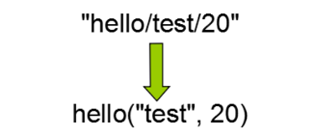C++11的实现思路如下：

1. 解析url将参数分割，分割之后的字符串放到列表中{"hello", "test", "2"}；

2. 将列表中的参数转换为function的实参；

2-1. 将列表中的参数分别转换为function的实参。

2-2. 将转换后的实参一个一个保存到“容器”--tuple中。

2-3. 将tuple转换为函数实参。

3. handler调用。

【字符串转换为对应的函数实参】

 `template::arity> struct invoker { template static inline void apply(const Function& func, token_parser & parser, Args const & args) { typedef typename function_traits::template args::type arg_type; router::invoker::apply(func, parser, std::tuple_cat(args, std::make_tuple(parser.get()))); } }; template struct invoker { template static inline void apply(const Function& func, token_parser &, Args const & args) { //参数列表已经准备好，可以调用function了 call(func, args); } };`

【实现函数调用】

 `template static void call_helper(F f, IndexTuple, const std::tuple& tup) { f(std::get(tup)...); } template static void call(F f, const std::tuple& tp) { call_helper(f, typename MakeIndexes::type(), tp); } `

C++11实现的http router不仅仅可以用于http服务器端的路由，还可以实现rpc和mvc框架的路由，所以用C++11去做web开发也是完全可以的。1500 次浏览  评价: 好 中 差订阅捐助

 深度解析：清理烂代码 如何编写出拥抱变化的代码 重构-使代码更简洁优美 团队项目开发"编码规范"系列文章
 重构-改善既有代码的设计 软件重构v2 代码整洁之道 高质量编程规范
 基于HTML5客户端、Web端的应用开发 HTML 5+CSS 开发 嵌入式C高质量编程 C++高级编程

 Visual C++编程命名规则 任何时候都适用的20个C++技巧 C语言进阶 串口驱动分析 轻轻松松从C一路走到C++ C++编程思想

 C++并发处理+单元测试 C++程序开发 C++高级编程 C/C++开发 C++设计模式 C/C++单元测试

 北京 嵌入式C高质量编程 中国航空 嵌入式C高质量编程 华为 C++高级编程 北京 C++高级编程 丹佛斯 C++高级编程 北大方正 C语言单元测试 罗克韦尔 C++单元测试每天2个文档/视频 扫描微信二维码订阅
 订阅技术月刊 获得每月300个技术资源